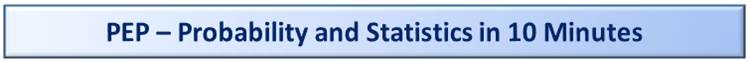Probability in 10 Minutes#1

Overview

This is Part 2 of 3 for what you might find in a typical Intro to Probability and Statistics course.

This covers topics related to Probability, Events, Intersection, Union, Mutually Exclusive, Permutations and Combinations.

Click this link for Part 1: Looking at Data in 10 Minutes

Click this link for Part 3: Statistics in 10 Minutes

Who Is This For?

Someone without any prior experience, such as a college freshman about to take her first Statistics class.

Key Goals for Each of the 3 Parts

1) Introduce the Concepts, Terminology and Symbols that would be covered in a typical Intro to Statistics course.

2) Provide an intuitive understanding of things versus just providing formulas.

3) Present the information step-by-step in the best order for learning.

PROBABILITY

Items Used in the Examples

Some of the examples may use these familiar items#2.

1) Coin Toss, Heads or Tails

2) Dice Roll, Six-sided Die

3) Deck of Cards, Standard 52 Card Deck

Probability Part 1: Probability and Events

1) Probability:  The odds that something will happen.  Expressed as a percent from 0 to 100% or as a decimal from 0 to 1.

Examples:

1.1) The probability of a coin toss landing on Heads is 50% or 0.50.

1.2) The probability of a dice roll being a 3 is 1/6 = 16.6666% or 0.166666.

2) Event: A single probabilistic activity like a dice roll or selecting a card from a deck.

We’ll use capital English letters like A, B, and C to denote an event.

And we’ll use P(E) to indicate the Probability of an Event happening.  ‘P’ for Probability and ‘E’ for a generic Event.   We’ll replace ‘E’ with letters like A, B, C, etc., for specific examples.

For Examples:

A = Pick a Card and it is a Heart.     P(A) = 13/52 = 25%

B = Pick a Card and it is a 9.     P(B) = 4/52 = 1/13 = 7.6923%

C = Roll a Die and get a number 2 or less.  P(C) = 2/6 = 33.3333%

To denote the opposite of an event, we’ll add an apostrophe.

Example:

A’ = Pick a card that is not a heart

A’ is pronounced “Not A” and is sometimes called a ‘Complementary#3 Event’.

An alternate symbol for A’ is to put a bar over the letter like Ā

Note that:

P(A) + P(A’) = 100%

Meaning that the probability of something happening plus the probability of that thing not happening adds up to 100%

Probability Part 2: Events: Mutually Exclusive, Overlapping, Union and Intersection

1) Mutually Exclusive:  Two Events that can’t happen at the same time.

Consider these example Events

A = Roll a 3.    Probability = 1/6 = 16.6666%

B = Roll an Even Number, i.e., 2, 4 or 6. Probability = 3/6 = 50%

C = Roll an Odd Number, i.e., 1, 3 or 5. Probability = 3/6 = 50%

A and B are Mutually Exclusive events.

A and C are not Mutually Exclusive events, meaning both can happen. That doesn’t mean both will happen, just that they can.

2) Overlapping:  Events are Overlapping if they have items in common.  Also known as ‘Joint’.  This is the opposite of Mutually Exclusive.

Example:

A and C from the above are Overlapping since they both have ‘3’ in common.

Another Example

D = Pick a card that is a Heart. P(D) = 13/52 = 25%

E = Pick a card that is a 3. P(E) = 4/52 = 7.6923%

D and E are overlapping since one of the 3s is also a Heart, meaning that both can happen.

3) Union:  In probability the Union of two events is the odds that either or both happen.  In logic, this would be an “OR”.

Symbol: U

This is an easy symbol to remember since it looks like a ‘U’ for ‘Union’.

P(A or B) = P(A U B)

To solve for the probability of A or B happening since A and B are Mutually Exclusive Events you add up the probabilities of each.

P(A U B) = P(A) + P(B)

P(A U B) = 16.6666% + 50%

P(A U B) = 66.6666%

That result should match our intuition as we are talking about the odds of rolling one of a 3 or a 2 or 4 or 6.  That is 4 out of the 6 possible rolls = 4/6 = 66.6666%

And remember, this formula only works when A and B are mutually exclusive. See below for a more generic formula that works in all cases.

4) Intersection:  In probability the Intersection of two events is the odds that both of them happen.  In logic, this would be an “AND”.

Symbol:

P(D and E) = P(D E)

For our example, this would be picking the 3 of Hearts, odds are 1/52 = 1.9231%

5) Generic Formula for the Probability of the Union of Two Events

To solve for the probability of D or E happening since D and E are Overlapping Events you add up the probabilities of each individually and then subtract the Intersection.

Looks like this:

P(D U E) = P(D) + P(E) - P(D E)

Remember that P(D U E) is the odds of either or both of D and/or E happening, either you draw a Heart or a 3 or both (which would be just drawing the 3 of Hearts).

We get:

P(D U E) = 13/52 + 4/52 – 1/52

P(D U E) = 25% + 7.6923% – 1.9231%

P(D U E) = 16/52

P(D U E) = 30.7692%

This formula also works with events that are Mutually Exclusive like A and B.  The Intersection of A and B is Zero, by definition of Mutually Exclusive, i.e., P(B B) = 0%

We get:

P(A U B) = P(A) + P(B) - P(B B)

P(A U B) = 16.6666% + 50% + 0%

P(A U B) = 66.6666%

Which is the same Probability as before.

Probability Part 3: Independent Events

1) Independence:  If one outcome of one event does not affect the outcome of another.

Example:

A = Flip a Coin and Get a Head.  P(A) = 1/2 = 50%

B = Pick a Card and have it be a Club.  P(B) = 13/52 = 25%

For Independent Events, to get the probability that they both happen, you multiple the probabilities of each.  Recall that the term that means that both events happen is Intersection.

For A and B as Independent Events:

P(A B) = P(A) * P(B)

P(A B) = 50% * 25%

P(A B) = 12.5%

In other words, if you flip a coin and pull a card, the probability that the coin lands on Heads and the card will be a Club is 12.5%.

If you flip a coin two times in a row, the second time is assumed to be Independent from the first time. i.e., whether the first toss was Heads or Tails, the probability of the second toss being a Head is still 50%.

2) Importance of Independence:  Whether or not two events are Independent has significance in many fields.

Example:

In finance, whether a particular stock price is independent or not to the price of Crude Oil can have important implications for investors#4.

Probability Part 4: Conditional Probability

1) Conditional Probability:  When knowing that one Event has occurred changes the probability that another Event has or will occur#5.

Symbol: |

This is read as ‘given’ and is written, for example like:

P(A | B) = The Probability of A given that B happened

P(B | A) = The Probability of B given that A happened

Example:

A = Roll a Die and have it be a 3.  P(A) = 1/6 = 16.6666%

B = Roll a Die and have it be an odd number.  P(B) = 3/6 = 50%

If someone Roll a Die and tells you that it landed on an odd number, what are the odds that it was a 3?

There are three odd numbers, 1, 3, and 5.   So the probability is 1/3 = 33.3333%

This is written as:

P(A | B) = 33.3333%

2) Formula for Conditional Probability

In the above, we calculated the probability by intuition.  Here is a formula that produces the same result:

P(A | B) = P(A B) / P(B)

This is valid only if P(B) > 0.

And recall that P(A B) is the Intersection of A and B, meaning the probability that they both happened.  In the above example, that would be 1/6 = 16.6666%, which is just the case were a 3 is rolled, i.e., if a 3 is rolled, the both A and B happened.

For the formula, the reverse is true as well, i.e., if P(A) > 0:

P(B | A) = P(A B) / P(A)

Probability Part 5: Bayes’ Theorem#6

We’ll explain Bayes’ Theorem, also known as Bayes’ Law starting with an example and then we’ll provide a generic formula.

Example:

Suppose you have 3 Factories. And let’s suppose they make ‘Widgets’.  A ‘Widget’ is whatever you want to be.  E.g., this could be a toy factory.

We’ll call the factories F1, F2, and F3.

These are the daily production numbers:

F1: 1000 Widgets

F2: 2000 Widgets

F3: 3000 Widgets

For a total of 6000 Widgets for the day.

Suppose that at the end of the day all production gets combined into one warehouse such that you don’t know which Widget came from which Factory.

Without yet getting to Bayes’ Theorem, we can say that if you randomly pull a Widget from the warehouse, these are the probabilities that it came from each of the three Factories:

P(F1) = 1000/6000 = 16.6666%

P(F2) = 2000/6000 = 33.3333%

P(F3) = 3000/6000 = 50.0000%

Which, as a double check, sums up to 100%

Next, we assume some rate of defects for each unit per factory, which we’ll express as a probability like this:

P(Error for a Widget Made in F1) = 1%

P(Error for a Widget Made in F2) = 2%

P(Error for a Widget Made in F3) = 3%

Again, note that we simply assumed those error rates.  i.e., they are assumptions, not calculations.

Let’s rewrite the above using our notation for Conditional Probabilities like this, using ‘E’ for ‘Error’

P(E | F1) = 1%

P(E | F2) = 2%

P(E | F3) = 3%

The first one in the list above is read as, ‘The Probability of an Error given that the Widget is made in Factory One is 1%’

Now let’s calculate the number of Errors for the day, per factory, based on the daily volume numbers and the percent error numbers, like this:

Number of Errors F1 = 1000 * 1% = 10 Widgets

Number of Errors F2 = 2000 * 2% = 40 Widgets

Number of Errors F3 = 3000 * 3% = 90 Widgets

That sums up to 140.  140 Defective Widgets in total for the day’s production.

Just by intuition we can see that *if* we select a random Widget *and* it has an error, then it is most likely from Factory 3.  That is because more defective Widgets come from Factory 3 than from either of the other 2 Factories.

Next, let’s write out the above using our notation:

P(F1 E) = P(F1) * P(E|F1)

The probability that a Widget was from Factory 1 and has an error equals the probability that the Widget came from Factory 1 times the probability of a Widget having an error given that it game from Factory 1.

Working this out to get the probability:

P(F1 E) = P(F1) * P(E|F1)

P(F1 E) = 16.6666% * 1%

P(F1 E) = 0.1667%

For all three Factories:

P(F1 E) = 16.6666% * 1% = 0.1667%

P(F2 E) = 33.3333% * 2% = 0.6667%

P(F3 E) = 50% * 3%         = 1.5000%

As a double check, you’ll get the same probabilities if you just take the number of errors from a given Factory divided by the total number of items produced:

For F1: 10 Widgets / 6000 Widgets = 0.1667%

For F2: 40 Widgets / 6000 Widgets = 0.6667%

For F3: 90 Widgets / 6000 Widgets = 1.5000%

For the overall Probability of Error for the day, we can use this formula:

P(Overall Error Rate) = Sum of Total Defective Widgets / Total Widgets

P(Overall Error Rate) = 140 / 6000

P(Overall Error Rate) = 2.3333%

Alternately, we could have just summed up the error rates for each Factory to get the same value.  We can do this because we are assuming they are Mutually Exclusive.

P(Overall Error Rate) = P(F1 E) + P(F2 E) + P(F3 E)

P(Overall Error Rate) = 0.1667% + 0.6667% + 1.5000%

P(Overall Error Rate) = 2.3333%

Same probability as before

Now after all of that background, we are at the part where we will ask the question that Bayes’ Theorem is designed to answer:

Question:

What is the probability that *given* that we found an error, that it came from a particular factory, e.g., F1.

That is written as:

P(F1|E) = ???

‘The probability that the Widget was produced by Factory 1 given that we saw an Error’

Remember our above description for Conditional Probability:

P(A | B) = The Probability of A given that B happened

In this example:

‘A’ = ‘Widget Produced in Factory 1’

‘B’ = ‘There is an Error/Defect’

We’ll start by calculating this using an intuition-based approach and then use the Bayes’ Theorem formula.

We can calculate the Probability by taking the number of Errors for a given Factor and dividing it by the total number of Errors:

P(F1 | E) = 10 Widgets / 140 Widgets = 7.1429%

P(F2 | E) = 40 Widgets / 140 Widgets = 28.5714%

P(F3 | E) = 90 Widgets / 140 Widgets = 64.2857%

And we can sanity check those values by seeing that they add up to 100%.  I.e., given that we found an error, we know it must have come from one of the three factors, i.e., 100% probability.

And now, finally, writing this out as Bayes’ Theorem for Factory 1.

P(F1 | E) = P(F1 E) / P(E)

“The probability that the Widget was produced by Factory 1 given that we saw an Error equals the probability that a Widget was produced at Factory1 that had errors (Intersection) divided by the Overall Probability of there being an Error”.

For all Factories, we get:

P(F1 | E) = P(F1 E) / P(E) = 0.1667% / 2.3333% = 7.1429%

P(F2 | E) = P(F2 E) / P(E) = 0.6667% / 2.3333% = 28.5714%

P(F3 | E) = P(F3 E) / P(E) = 1.5000% / 2.3333% = 64.2857%

Let’s highlight the below difference for increased clarity:

This:

1) “The probability that a random Widget came from Factory 3”.

Is not the same as:

2) “The probability that a Widget *that was defective* came from Factory 3”.

For Number 1 above, that is written as P(F3) and the probability is:

[3000 Widgets Produced by Factory 3] / [6000 total Widgets] = 50%.

For number 2 above, that is written as P(F3|E) and the probability is, as shown: 64.2857%

You may see Bayes’ Theorem written this alternate way:

P(F1 | E) = [P(E | F1) * P(F1) ] / P(E)

And just recall that we previously wrote that:

P(F1 E) = P(F1) * P(E|F1)

Or, to switch the two terms to the right of the equals sign

P(F1 E) = P(E|F1) * P(F1)

In other words both of these formulas are variations of Bayes’ Theorem.

P(F1 | E) = P(F1 E) / P(E)

or use

P(F1 | E) = [P(E | F1) * P(F1) ] / P(E)

Lastly, you’ll likely see this formula written out with ‘A’ and ‘B’, generically, which we’ll provide for completeness.

P(A | B) = P(A B) / P(B)

or use

P(A | B) = [P(B | A) * P(A) ] / P(B)

A = Widget Produced at Factory

B = Widget Has an Error/Defect

Keeping in mind the requirement that P(B) must not be zero, i.e., dividing by zero is not allowed.

In summary, with good intuition you can probably figure out the correct answer to the type of question that Bayes’ Theorem is designed to answer, meaning without using the formula.  Or you can use the Bayes’ Theorem formula, so long as you have clarity on what is meant by each of the terms, which includes the concepts of Conditional Probability and Intersection.

Probability Part 6: Permutations and Combinations

1) Permutation:  An arrangement of a number of items in a particular order.  Order Matters.

2) Combination: Similar to a Permutation, except that order does not matter.

3) Factorial: This is a mathematical concept that is defined as:

n! = n(n-1) * n(n-2) …  (3) * (2) * (1)

n! is read as ‘n factorial’

where ‘n’ can be any whole number like 1, 2, 3, 4, etc.

in addition, by definition, we say that

0! = 1

Which is read as zero factorial equals one.

For example, 6! is:

6! = 6 * 5 * 5 * 4 * 3 * 1

The formula in Excel is ‘FACT’, e.g.,:

=FACT(6)

n! is also equivalent to n * (n-1)!

e.g., for 6, that is:

6! = 6 * 5!

You can be more generic like

n! = n * (n-1) * (n-2) * (n-3)!

e.g.,

6! = 6 * 5 * 4 * 3!

Factorial and the properties of factorials as described above are useful tools working with Permutations and Combinations.

4) Permutation Example for a Specific Number of Items

Example

If you have 5 balls, all of a different color, how many different ways can you arraign them?

5! = 5 * 4 * 3 * 2 * 1 = 120

Showing as an example for a smaller number of items, if you have 3 balls, red, green, blue, that is 3! = 3 * 2 * 1 = 6 permutations like:

Permutation 1: RGB

Permutation 2: GRB

Permutation 3: BRG

Permutation 4: BGR

Permutation 5: RBG

Permutation 6: GBR

5) Permutation Example When You Take Some Subset of Items.

Consider taking ‘r’ items out of a total available of ‘n’.    That could be, for example, taking 4 balls out of a set of 6 balls, with each of the 6 balls being a different color.

e.g., if the colors are red, green, blue, white, black and orange, then one of the permutations of 4 balls would be, for example, white, black, red, blue.  i.e., 4 of the 6.

This case is written as

nPr

which in this example is:

6P4

i.e., taking ‘r’ items at a time from a set of ‘n’.  In this example, taking 4 items from a set of 6.

‘r’ must be a value the same or less than ‘n’.  e.g., if ‘r’ is the same as ‘n’, then you can take 6 out of 6 items, but you can’t take 7 out of 6 items.

The generic formula is:

nPr  = n! / (n - r)!

so for our example of the 4 balls from 6, we have:

nPr  = 6! / (6 - 2)!

nPr  = 6! / 2!

nPr  = (6 * 5 * 4 * 3 * 2 * 1) / (2 * 1)

nPr  = 360

5) Combinations Example

This example will be added soon

Footnotes

#1) The title of this page was inspired by ‘Learn Python in 10 Minutes’ at:

#2) Items were chosen for the examples with the expectation that they are familiar to most readers. Here is more information for people who need it:

2.1) Coin Toss

Tails = 1 (one)

2.2) Dice Roll - Dice is Plural, Singular is ‘Die’.

Single six-sided Die, with numbers 1 to 6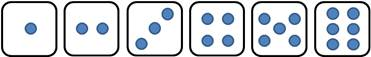2.3) Deck of Cards

Standard deck with 52 cards

With four Suits:

Hearts (Red)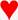Diamonds (Red)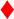Clubs (Black)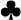Spades (Black)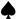And each suit having cards numbered 1 to 13 for a total of 4 * 13 = 52 cards.

An Ace = 1, then cards numbered 2 to 10, then a Jack = 11, a Queen = 12 and a King = 13.

#3) As a way remember ‘complement’ which means the opposite of something and ‘A Compliment’ which means saying something positive about someone, use this mnemonic:

‘I like compliments’.

The I in ‘I Like’ will remind you that compliment, i.e., saying something nice about someone, has an ‘I’ in it.

#4) The statistics term frequently used in Finance, i.e., financial markets like stocks, bonds and commodities is ‘Correlation’.  Correlation is a measure of how two things move together.  e.g., two stocks or one stock and the price of gold.

Items could be Positively Correlated: meaning when one of the two goes up, the other is likely to go up as well.

Items could be Negatively Correlated: meaning when one of the two goes up, the other is more likely to go down and vice-versa.

Items could be Uncorrelated: This is also called ‘not correlated’.  This is equivalent to saying that both items are ‘Independent’ based on our terminology. When two items are Uncorrelated (i.e., Independent) known that one of them when up or down in price doesn’t give you any information as to whether or not it is likely that the other went up or down in price.

#5) A common example of Conditional Probability is estimated life span for people.  For example, in one country, a person at birth may be predicted to live until 80. However, that would not be the case for someone who is already 75.  For someone who is already 75, they might be expected to live until 85.

You would say, given that the person already lived to be 75, they are expected to live another 10 years until 85.

As a more extreme example to help make things more intuitive, if the average life expectancy for a newborn is 80 years, what would you think for someone already 81 years old?  You wouldn’t expect them to immediately drop dead, as being one year after their expected lifespan.  The key is to realize that the 80 years estimate is for newborns and not someone who has, in this case, already reached 81.   The 81 year old might be expected to live until 88.

#6) With regard to Bayes’ Theorem, this is not necessarily going to be part of an Intro to Statistics course.  That said, I figured it was better to include it here versus to not include it.  If you are not learning Bayes’ Theorem in your class, then you can skip this section.